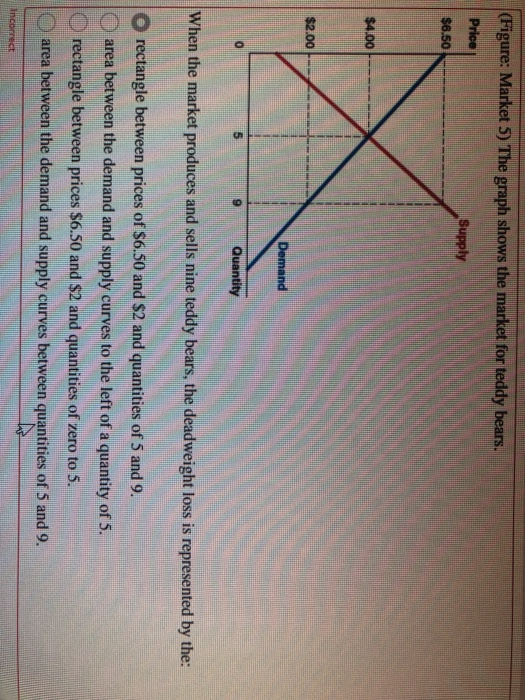# Question (Figure: Market 5) The graph shows the market for teddy bears. Price Supply $6.50$4.00 $2.00 Demand Quantity When the market produces and sells nine teddy bears, the deadweight loss is represented by the: rectangle between prices of$6.50 and $2 and quantities of 5 and 9. area between the demand and supply curves to the left of a quantity of 5. rectangle between prices$6.50 and $2 and quantities of zero to 5. area between the demand and supply curves between quantities of 5 and 9. IncorrectLWG5W7 The Asker · EconomicsTranscribed Image Text: (Figure: Market 5) The graph shows the market for teddy bears. Price Supply$6.50 $4.00$2.00 Demand Quantity When the market produces and sells nine teddy bears, the deadweight loss is represented by the: rectangle between prices of $6.50 and$2 and quantities of 5 and 9. area between the demand and supply curves to the left of a quantity of 5. rectangle between prices $6.50 and$2 and quantities of zero to 5. area between the demand and supply curves between quantities of 5 and 9. Incorrect
More
Transcribed Image Text: (Figure: Market 5) The graph shows the market for teddy bears. Price Supply $6.50$4.00 $2.00 Demand Quantity When the market produces and sells nine teddy bears, the deadweight loss is represented by the: rectangle between prices of$6.50 and $2 and quantities of 5 and 9. area between the demand and supply curves to the left of a quantity of 5. rectangle between prices$6.50 and \$2 and quantities of zero to 5. area between the demand and supply curves between quantities of 5 and 9. Incorrect